# Questions pairs identification

Source: Deep Learning on Medium

There are no stupid questions…only duplicates!

### Background

You have a burning question — you login to Quora, post your question and wait for responses. There is a chance that what you asked is truly unique but more often than not if you have a question, someone has had it too. Did you notice that Quora tells you that a similar question has been asked before and gives you links directing you to it? How does Quora detect that the question you just asked matches with the other questions already asked before?

Intrigued by this question, my team — Jui Gupta, Sagar Chadha, Cuiting Zhong and I decided to work on the Kaggle Quora duplicate questions challenge. The goal was to use sophisticated techniques to understand question semantics and highlight duplicate (similar) questions.

But why would a company want to highlight duplicate questions?

1. Cheaper data storage — Storing less questions! Obviously!
2. Improved Customer Experience — Faster responses to questions.
3. Re-use content — If a question has been answered before, it is very efficient to use the same answer for a similar question.

### The Data

The data set consisted of around 400,000 pairs of questions organized in the form of 6 columns as explained –

id: Row ID

qid 1, qid 2: The unique ID of each question in the pair

Question 1, question 2: The actual textual contents of the questions.

is_duplicate: Label is 0 for questions which are semantically different and 1 for questions which essentially would have only one answer (duplicate questions). 63% of the questions pairs are semantically non-similar and 37% are duplicate questions pairs.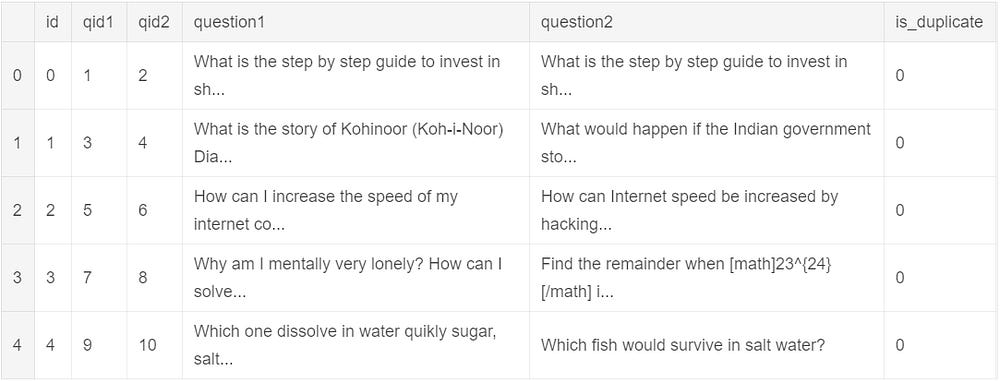A look at the data

#### Dataexploration

An analysis of the data showed the most common words in the questions were the following –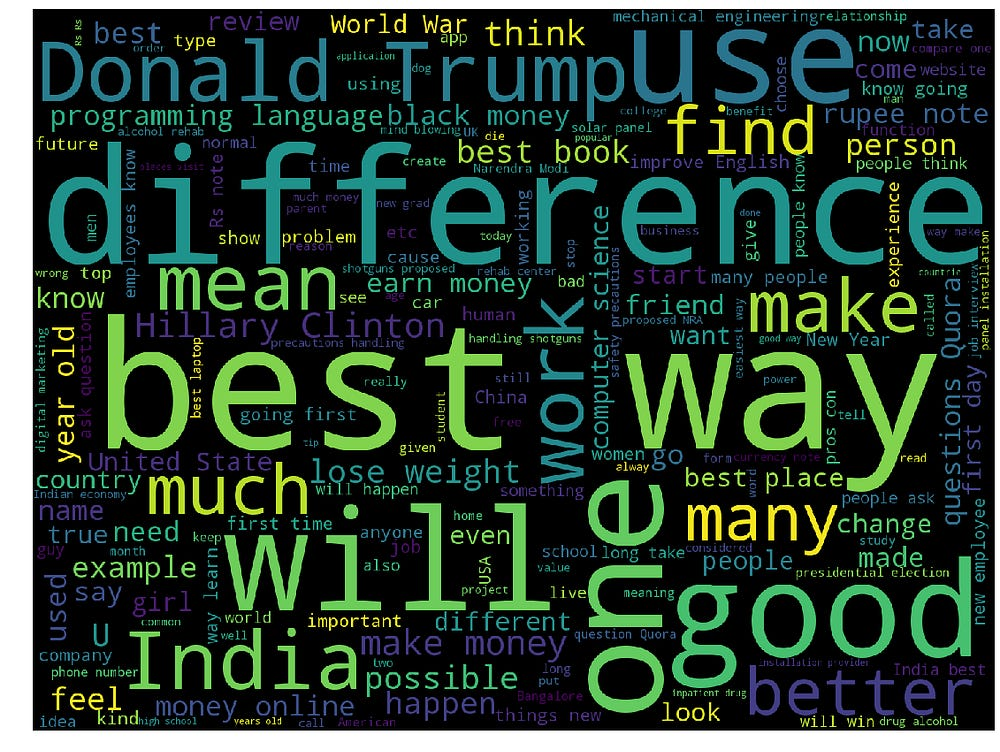Word cloud for most common words

Duplicate questions marked as not duplicate

We also found some question pairs that, although duplicate, were marked as 0 in the labels. Some of these are shown below –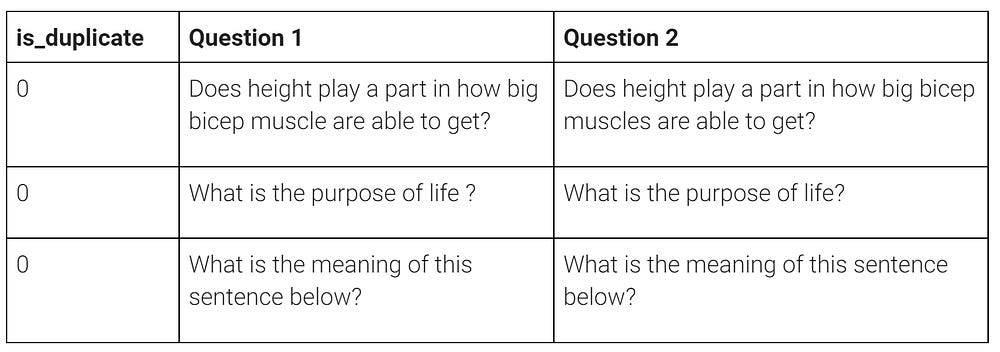Duplicates marked as different

The labels for these questions were changed to 1 to improve the accuracy of the model!

Modelling Approach

A very simple approach to detecting similarity between a pair of questions would be to look at unique words in the first question that are also present in the second question as a ratio of the total words in both questions. This number could then be used in a simple model such as logistic regression to predict duplicate versus different questions.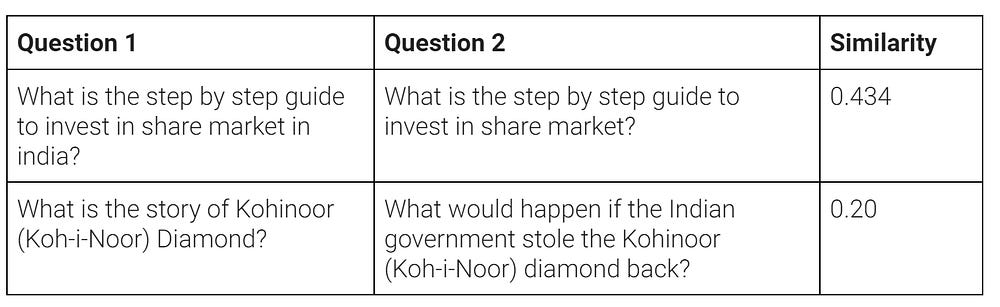Word Similarity between questions

This approach has limitations since two questions with very few words in common can still have the same meaning. This could be due to different sentence structures, use of synonyms, etc. Consider the sentences “What to do to be a data scientist” and “What qualities make a good data scientist”. While these have very few common words (exclude stopwords), the intent of the asker is the same. In order to go beyond comparing words in a sentence, we need a way to understand the semantic meaning of the questions in consideration.

#### Sentence Embedding

Generating sentence embedding is a three step process –

1. Sentence Tokenization — Using all the questions in our data, we create a large dictionary that maps each word to a unique integer index. This dictionary is then used to convert sentences from sequences of strings to sequences of integers.
2. Zero Padding — The next step in the process is to ensure that the input to the model (neural network) is of uniform length. To accomplish this, we chose a maximum length for each of the questions — 25 in our analysis— and then truncated or 0 padded the sentences to this length. 0s are inserted at the beginning of sentences that are fewer than 25 words in length.
3. Embedding matrix — Finally, we use a pretrained word embedding to convert each word into a vector representation. Each word is converted into a 300 long vector.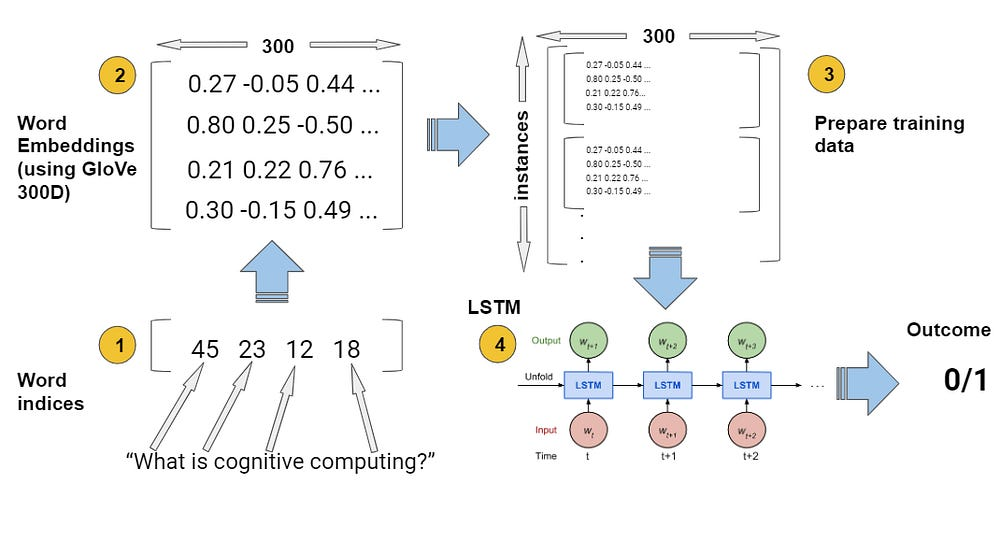Process to get sentence embedding

The process described above creates a data tensor from our text data of dimensions — (200000, 25, 300) for each of question 1 and question 2. This serves a dual purpose –

1. Converts text strings to numbers that can be used to train a neural network
2. Gives a representation of our data that encodes the meaning of and relationship between the words. Using simple mathematics, we can determine if two words are similar in meaning or completely opposite.

The data tensor so created is then sent through a neural network model for training which we describe below.

#### Bag of Embedding Approach

The embedding created using the methodology above is then to be passed through the network drawn above. Let’s see what is happening in the network above –

Time Distributed Dense Layers — These are used for temporal data when we want to apply the same transformation to every time-step. In our data set, each question has 25 words which correspond to 25 time steps. We use a dense layer with 300 hidden inputs — since our data has 300 dimensional embedding, we get 90,000 + 300 (bias) = 90,300 weights for the layer. Both question 1 and question 2 pass through similar time distributed layers.

The below diagram makes clear the transformation —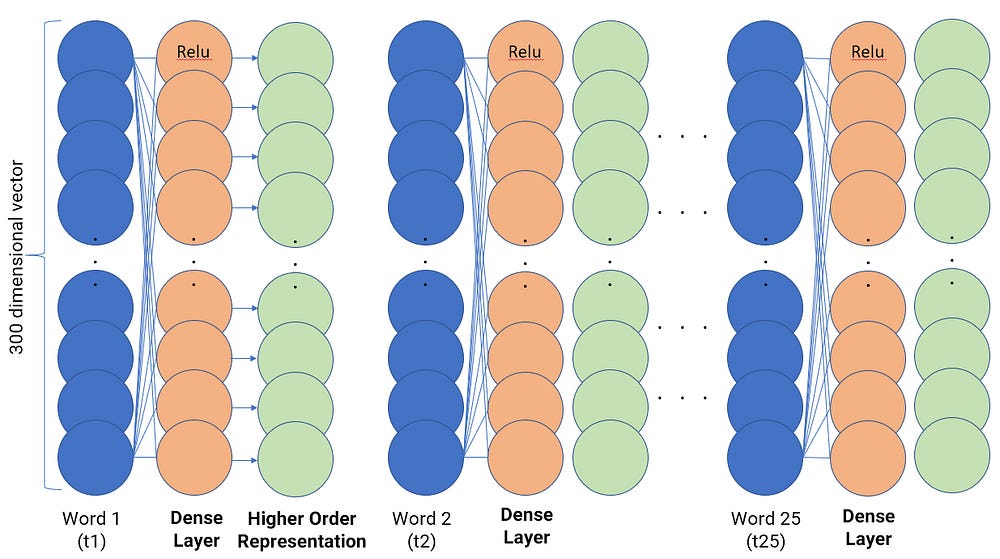Time Distributed Dense Layers

Each of the 300 hidden units in the time distributed dense layer (shown in orange) connect with the word vectors at each time step (shown in blue) and produce higher order representations (shown in green). All the dense layer units have the ‘Relu’ activation for non-linearity.

Lambda layers — Lambdas in Keras are like the ‘def’ keyword in python — they allow us to use custom layers in our model. We use the lambda layer on the higher order representations obtained after the time distributed dense layers to get an average sense of the meanings of all the words in the question.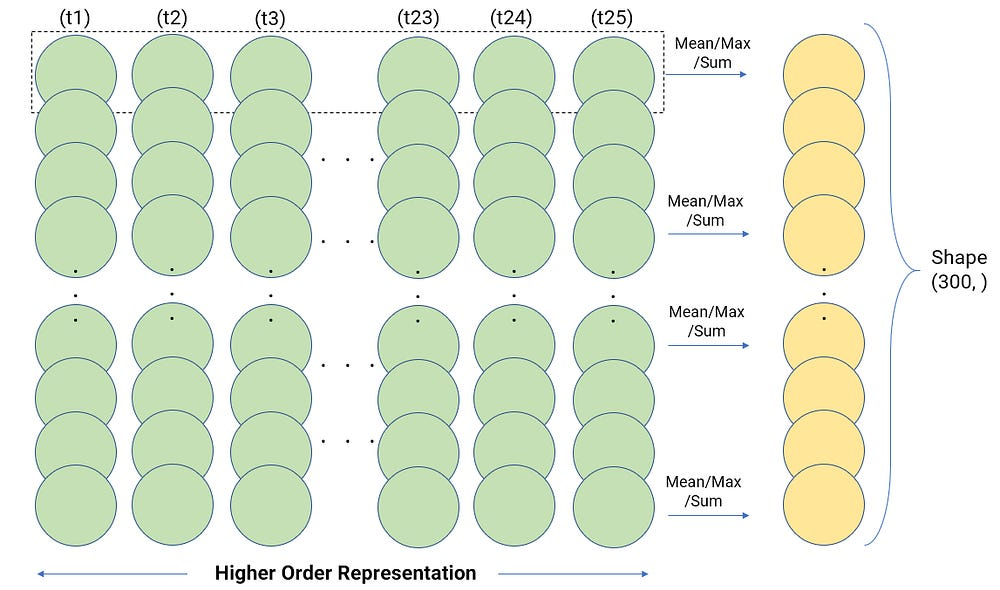Lambda layers

Computing the average, in essence, computes an aggregate representation of the question in 300 dimension. This encapsulates the meaning of the entire question in those dimensions. Average is just one of the possible aggregations, there are others possible aggregations such as max, sum, etc.

#### Bi-LSTM with Attention Approach

The simple bag of embedding model architecture mentioned above did achieve a pretty good accuracy. Then why bother to use Bidirectional LSTM and attention layer?

When we went back and manually checked the question pairs causing the highest mis-classifications, we found that it was mostly due to the longer sentences. This makes sense because we had not accounted for a way to capture and implement words in the previous and future state into our present state. This requires to implement an adaptive gating mechanism which is provided by networks like LSTMs. On researching we were lucky to find a paper on using Bidirectional LSTMs for relational classification, which was used in tasks like image captioning, question answering and so on.

Now coming to the model, the changes we made involved adding a Bidirectional LSTM after the word embedding stage to incorporate higher level features into out embedding vectors. After this, unlike before where we were concatenating the questions, we implement attention by carrying out similarity between the pairs.

Attention layer — Unlike the previous bag of words the attention layer involves calculation of a dot product between the questions followed by a dense layer without any non linearity.

After finishing modeling, how did we evaluate our models?

Since it was a binary classification task we used the binary cross entropy (log loss) to calculate our accuracy.

Binary cross entropy:

The baseline accuracy is 63% because that’s how our data was split. Here’s the model performance for models we built.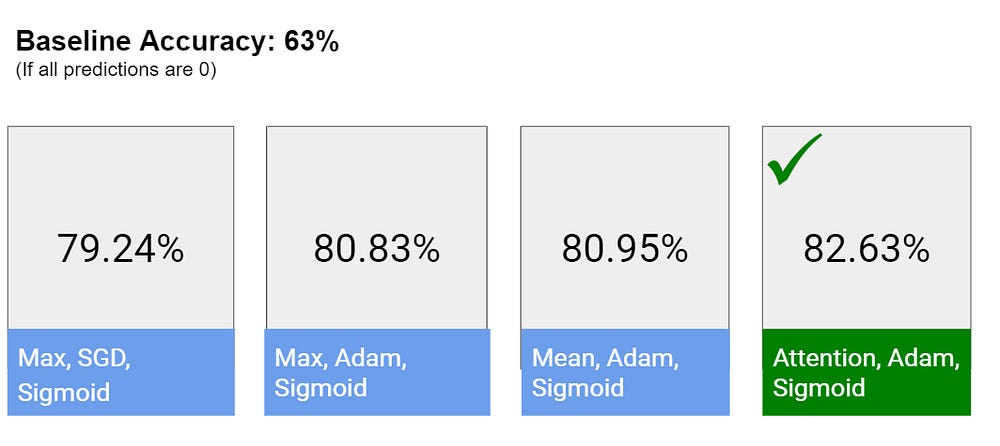Model accuracy for our analysis

Future Work

• Use different pretrained embeddings for the model. e.g. Word2Vec, fasttext
• Try different similarity measure in embedding concatenation. e.g. Manhattan distance
• Extract and combine other additional NLP features. e.g. number/proportion of common words
• Another interesting problem that utilizes the same concept is that of question answering using a context passage. We can attempt that.

### References

None of this work could have been done on our own. Check out the following references to get access to all the great resources we used:

Feel free to let us know what you think and ways we can improve upon what we have! :)